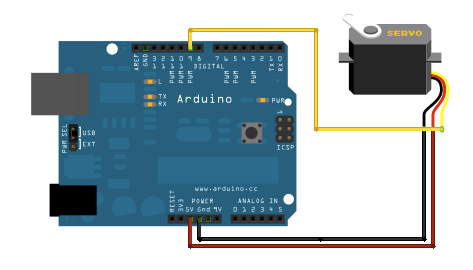Tutorial : MG995 Servo Motor### For this tutorial you will need

• MG995 Servo motor
• Arudino Uno• Red wire - 5v
• Brown - Ground
• Yellow - Digital Pin 9

Upload the code to arduino sketch

```#include <Servo.h>
Servo myservo;  // create servo object to control a servo
// a maximum of eight servo objects can be created
int pos = 0;    // variable to store the servo position
void setup() {
myservo.attach(9);  // attaches the servo on pin 9 to the servo object
}
void loop() {
for(pos = 0; pos < 180; pos += 1)  // goes from 0 degrees to 180 degrees
{                                  // in steps of 1 degree
myservo.write(pos);              // tell servo to go to position in variable 'pos'
delay(15);                       // waits 15ms for the servo to reach the position
}
for(pos = 180; pos>=1; pos-=1)     // goes from 180 degrees to 0 degrees
{
myservo.write(pos);              // tell servo to go to position in variable 'pos'
delay(15);                       // waits 15ms for the servo to reach the position
}
}```